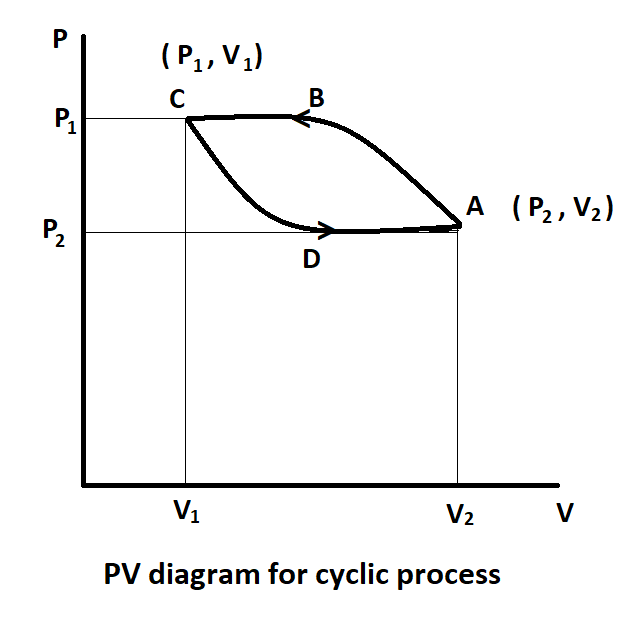For a cyclic process which of the following quantities is zero?A. $\Delta V$ B. $\Delta U$C. WD. $\Delta Q$Verified
148.5k+ views
Hint: To solve this question, we have to remember that any cyclic process has an initial state the same as the final state. So, if any quantity is zero in a cyclic process it means it depends on the state of the process and not on the process using which it has reached that state. So, find which of the given quantities is a state function to get the answer.